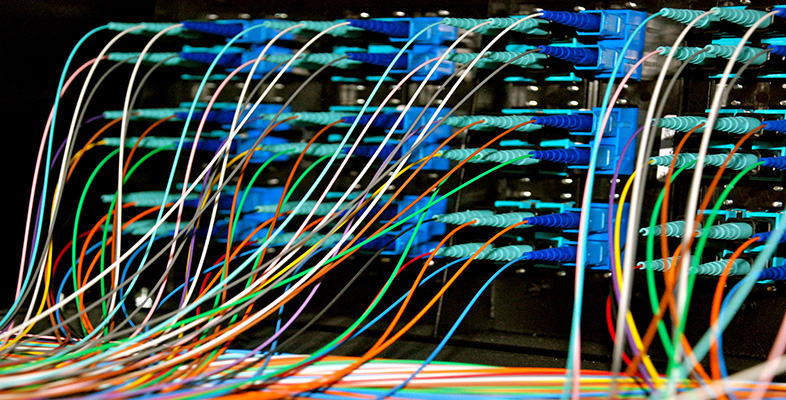Representing and manipulating data in computers

Start this free course now. Just create an account and sign in. Enrol and complete the course for a free statement of participation or digital badge if available.

Free course

# 6 Manipulating data in computers: introduction

Sections 1 to 5 of this course have shown that in a computer all types of data are represented by binary codes, and that programmers must make sure that the programs they write treat this data appropriately in any particular application: as text if it is intended to be text, as a binary fraction if it is intended to be a binary fraction, and so on.

Programmers must also ensure that the programs manipulate the binary codes in an appropriate way for the particular application. But what sorts of manipulation are possible inside a computer?

Perhaps surprisingly, a great deal of data manipulation in a computer is simply moving data around without changing it at all.

Not so surprisingly, a fair amount of the data manipulation takes the form of arithmetic: addition, subtraction, multiplication and division. For example, some of the lossy compression techniques I mentioned in Sections 3.3 and 4.3 require a great deal of arithmetic. I'll talk more about arithmetic involving binary numbers in Section 7.

Another common manipulation is comparing data (with a view to taking an action that depends on the result of the comparison). This is usually referred to as testing. Think for example about a spell-check program that is checking whether a word in the email you have just typed is in its dictionary. The letters in your word and the letters in the word in the dictionary will all be in ASCII format. Pairs of ASCII codes can then be compared systematically, and if the codes in each pair are identical then your word is identical to the word in the dictionary and the spell checker can move on to the next word in your email. If all the codes are not identical then either the computer has yet to find the word you are using, or possibly you have a made spelling mistake, or the word is not in its dictionary. Either way, what the computer does next depends on the outcome of the comparison test.

The fourth sort of data manipulation, not so common but still used, is logic operations on the data. I'll explain what I mean by logic operations in Section 8, and give an example of how such manipulations could be used.

So there are four basic types of data manipulation carried out in computers:

• moving data around unchanged;

• carrying out arithmetic operations on data;

• testing data;

• carrying out logic operations on data.

In this course I will focus on arithmetic operations (Section 7) and logic operations (Section 8).

In computers, arithmetic and logic operations are carried out by a crucial component in the processor called an arithmetic-logic unit (ALU). So when you look at arithmetic and logic operations in Sections 7 and 8 you will be looking at the operations carried out by the processor's ALU.

For simplicity, I shall use 8-bit words throughout Sections 7 and 8, but the principles are equally applicable to binary data words of other lengths, and ALUs may indeed be designed to act on 16-, 32- or even 64-bit words.